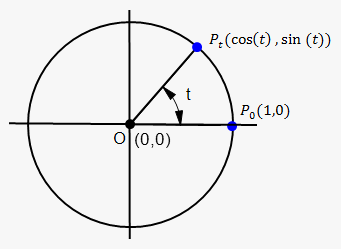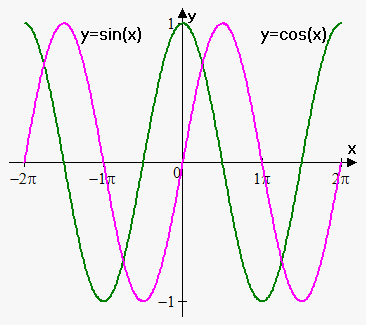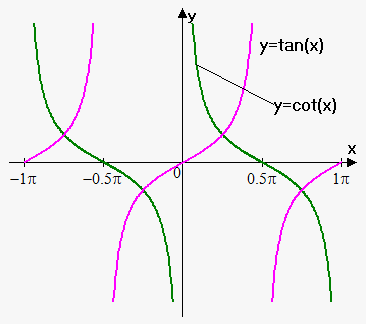Trigonometric FunctionsConsider unit circle centered at origin and point ${P}_{{0}}{\left({1},{0}\right)}$. If we begin to rotate point ${P}_{{0}}$ around origin on angle ${t}$ then we will obtain point ${P}_{{t}}$.

x-coordinate of this point is called cosine of number ${t}$ and denoted by ${\cos{{\left({t}\right)}}}$, y-coordinate of this point is called sine of number ${t}$ and denoted by ${\sin{{\left({t}\right)}}}$.

Tangent of number ${t}$ is ratio of sine and cosine: ${\tan{{\left({t}\right)}}}=\frac{{{\sin{{\left({t}\right)}}}}}{{{\cos{{\left({t}\right)}}}}}$.

Cotangent of number ${t}$ is ratio of cosine and sine: ${\cot{{\left({t}\right)}}}=\frac{{{\cos{{\left({t}\right)}}}}}{{{\sin{{\left({t}\right)}}}}}$.

Secant of number ${t}$ is ${\sec{{\left({t}\right)}}}=\frac{{1}}{{{\cos{{\left({t}\right)}}}}}$.

Cosecant of number ${t}$ is ${\csc{{\left({t}\right)}}}=\frac{{1}}{{{\sin{{\left({t}\right)}}}}}$.

When we talk about trigonometric functions we can use both radian and degree measure of angle ${t}$, but in calculus we almost always use radian measure (unless other stated).

To convert radian measure to degree and vice versa following formulas are used:

${1}\ {r}{a}{d}=\frac{{{{180}}^{{0}}}}{\pi}\approx{{57}}^{{0}}$ and ${{1}}^{{0}}=\frac{\pi}{{{{180}}^{{0}}}}\ {r}{a}{d}\approx{0.017}\ {r}{a}{d}$.

So, $\pi$ is ${{180}}^{{0}}$, ${2}\pi$ is ${{360}}^{{0}}$, $\frac{\pi}{{2}}$ is ${{90}}^{{0}}$ etc.

Domain of cosine and sine is ${\left(-\infty,\infty\right)}$, their range is ${\left[-{1},{1}\right]}$.These functions are periodic with main period ${2}\pi$, i.e. ${\sin{{\left({x}+{2}\pi\right)}}}={\sin{{\left({x}\right)}}}$ and ${\cos{{\left({x}+{2}\pi\right)}}}={\cos{{\left({x}\right)}}}$ for all ${x}$.

Domain of tangent function is all ${x}$ except those ${x}$ where ${\cos{{\left({x}\right)}}}={0}$.

Range of tangent function is ${\left(-\infty,\infty\right)}$.

Tangent is periodic function with period $\pi$: ${\tan{{\left({x}+\pi\right)}}}={\tan{{\left({x}\right)}}}$ for all ${x}$.Domain of cotangent function is all ${x}$ except those ${x}$ where ${\sin{{\left({x}\right)}}}={0}$.

Range of cotangent function is ${\left(-\infty,\infty\right)}$. Cotangent is periodic function with period $\pi$: ${\cot{{\left({x}+\pi\right)}}}={\cot{{\left({x}\right)}}}$ for all ${x}$.

Following formulas hold for trigonometric functions. They will be used in further notes:

1. ${{\cos}}^{{2}}{\left({x}\right)}+{{\sin}}^{{2}}{\left({x}\right)}={1}$ for all ${x}$.
2. ${1}+{{\tan}}^{{2}}{\left({x}\right)}={{\sec}}^{{2}}{\left({x}\right)}$ for all ${x}$.
3. ${1}+{{\cot}}^{{2}}{\left({x}\right)}={{\csc}}^{{2}}{\left({x}\right)}$ for all ${x}$.
4. ${\sin{{\left({x}\pm{y}\right)}}}={\sin{{\left({x}\right)}}}{\cos{{\left({y}\right)}}}\pm{\cos{{\left({x}\right)}}}{\sin{{\left({y}\right)}}}$ for all ${x},{y}$.
5. ${\cos{{\left({x}+{y}\right)}}}={\cos{{\left({x}\right)}}}{\cos{{\left({y}\right)}}}-{\sin{{\left({x}\right)}}}{\sin{{\left({y}\right)}}}$ for all ${x},{y}$.
6. ${\cos{{\left({x}-{y}\right)}}}={\cos{{\left({x}\right)}}}{\cos{{\left({y}\right)}}}+{\sin{{\left({x}\right)}}}{\sin{{\left({y}\right)}}}$ for all ${x},{y}$.
7. ${\tan{{\left({x}+{y}\right)}}}=\frac{{{\tan{{\left({x}\right)}}}+{\tan{{\left({y}\right)}}}}}{{{1}-{\tan{{\left({x}\right)}}}{\tan{{\left({y}\right)}}}}}$ for all ${x},{y}$.
8. ${\tan{{\left({x}-{y}\right)}}}=\frac{{{\tan{{\left({x}\right)}}}-{\tan{{\left({y}\right)}}}}}{{{1}+{\tan{{\left({x}\right)}}}{\tan{{\left({y}\right)}}}}}$ for all ${x},{y}$.

Trigonometric functions, because of periodicity, are widely used for modeling repetitive events: motion of pendulum, vibrating string, sound waves etc.